# NCERT Exemplar Class 11 Physics Solutions for Chapter 10 - Mechanical Properties Of Fluids

NCERT exemplar Class 11 Physics Chapter 10 Mechanical Properties of Fluids has questions from NCERT exemplar book along with questions from previous papers, HOTS, Numerical problems, worksheet on Mechanical Properties of Fluids, important formulas and all derivations of mechanical properties of fluids Class 11. Important questions of mechanical properties of fluids, NEET questions of mechanical properties of fluids prepared by experts at BYJU’S helps you to take mechanical properties of fluids NEET notes which helps you in mechanical properties of fluids revision. Fluids are substances that can flow easily and do not possess a definite shape. Understanding the mechanical properties of fluids is essential because an object can be submerged in a liquid can portray with different properties. The fluid exerts a force on its surface which is termed as the thrust of the liquid. Other important concepts that are introduced in this chapter are:

1. Pressure
2. Pascal’s Law
3. Law of Flotation
4. Archimedes Principle
5. Equation of Continuity
6. Bernoulli’s Theorem
7. Viscosity
8. Stoke’s Law

Check out the NCERT Exemplar Class 11 Physics Chapter 10 Mechanical Properties of fluids available as a PDF below:

## Download PDF of NCERT exemplar class 11 physics Chapter 10 Mechanical Properties of Fluids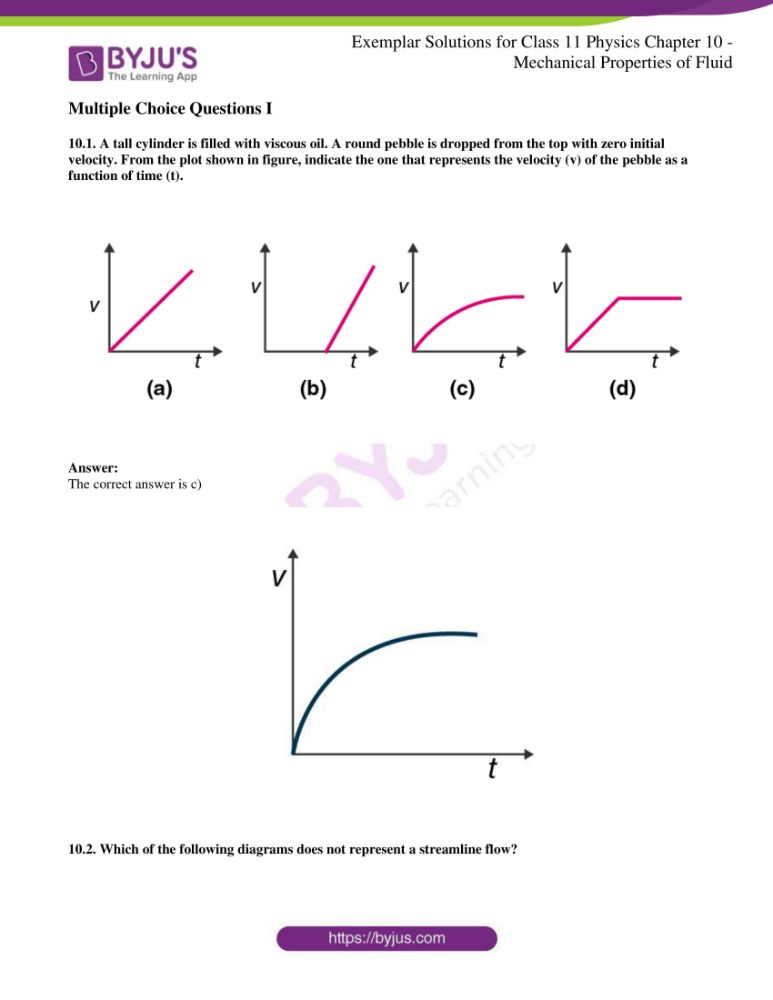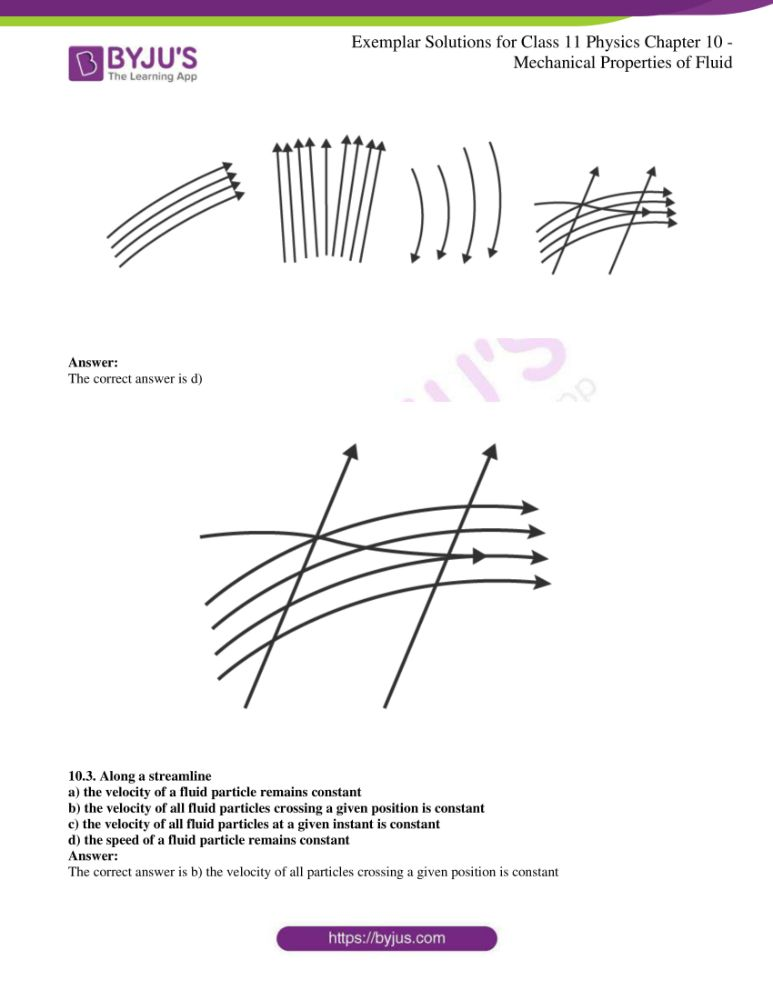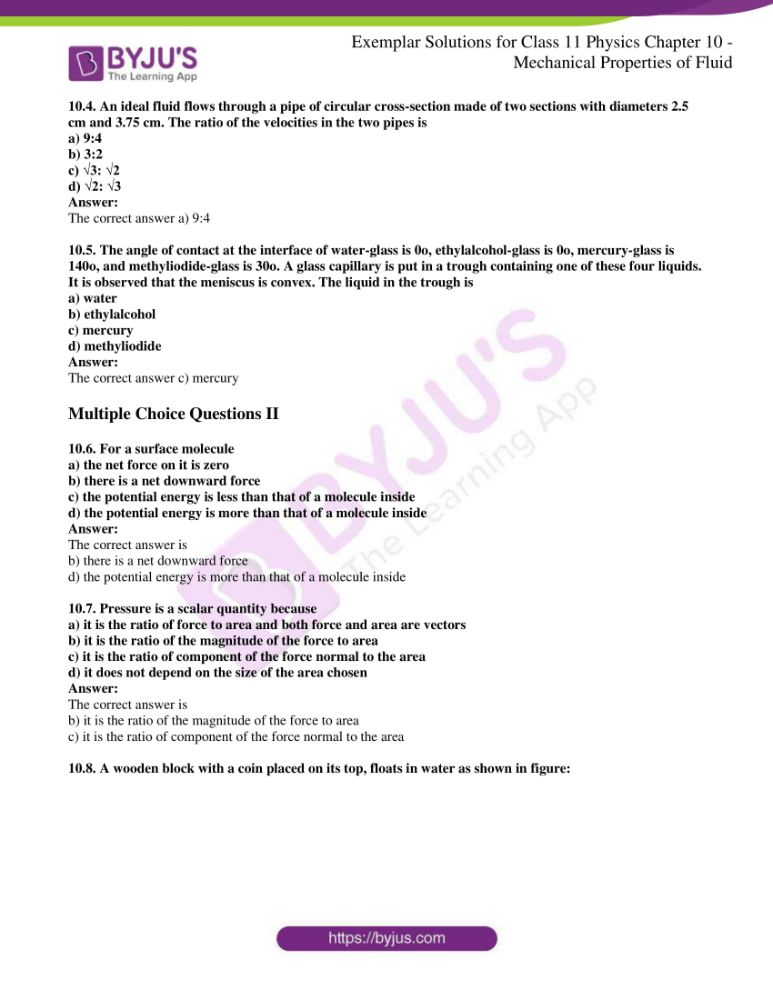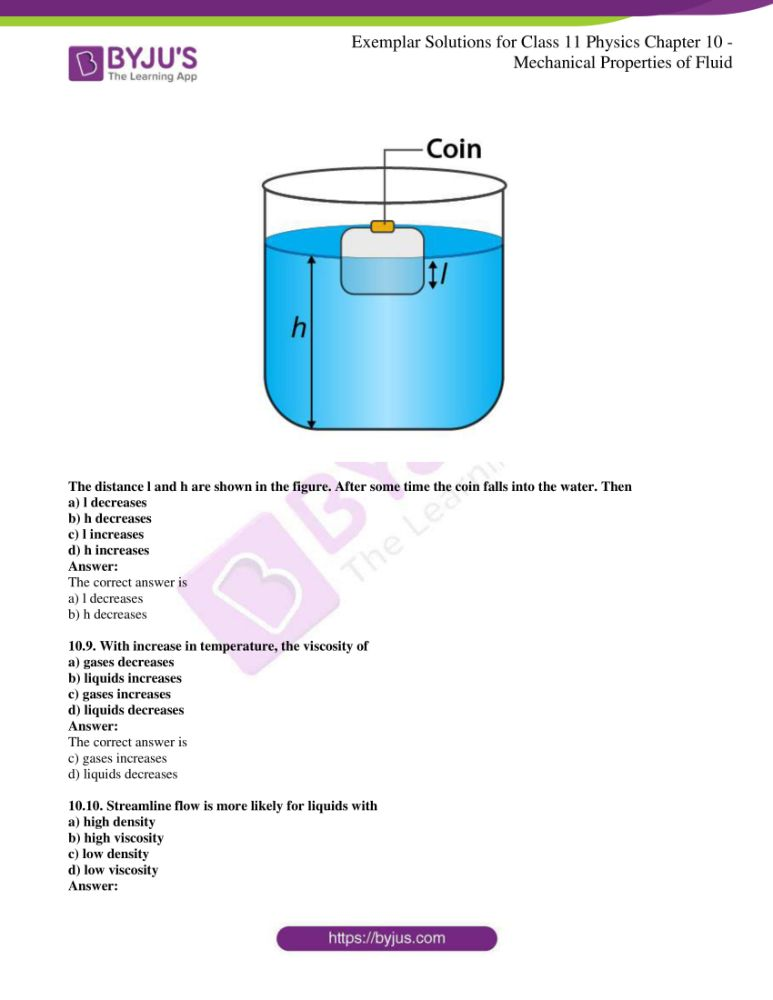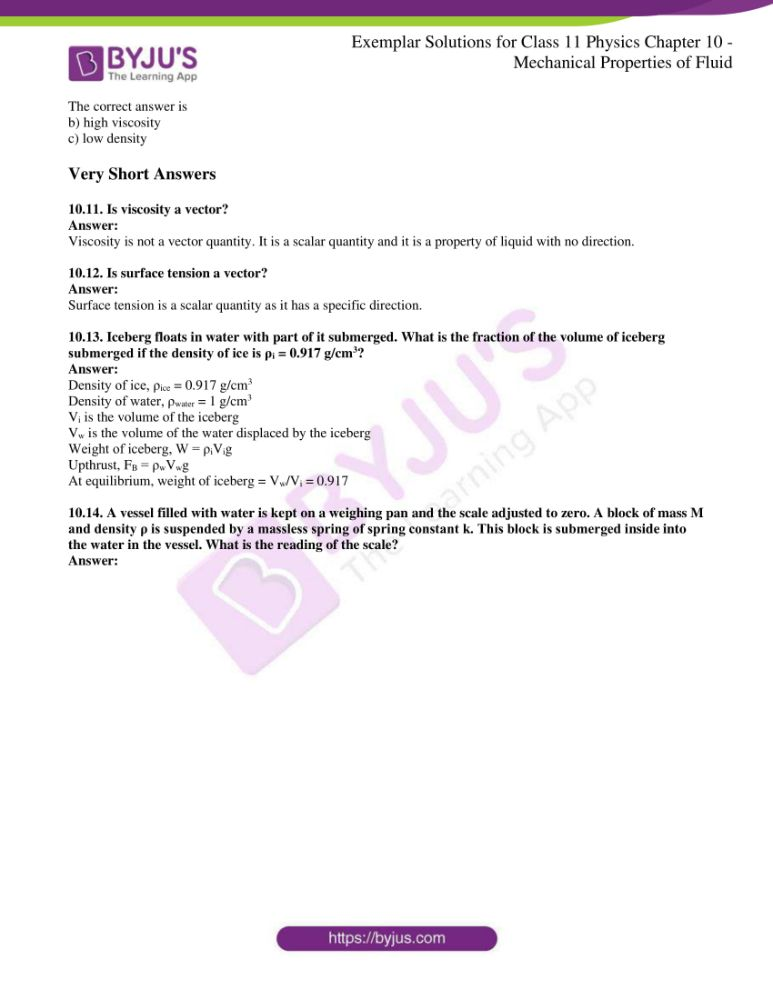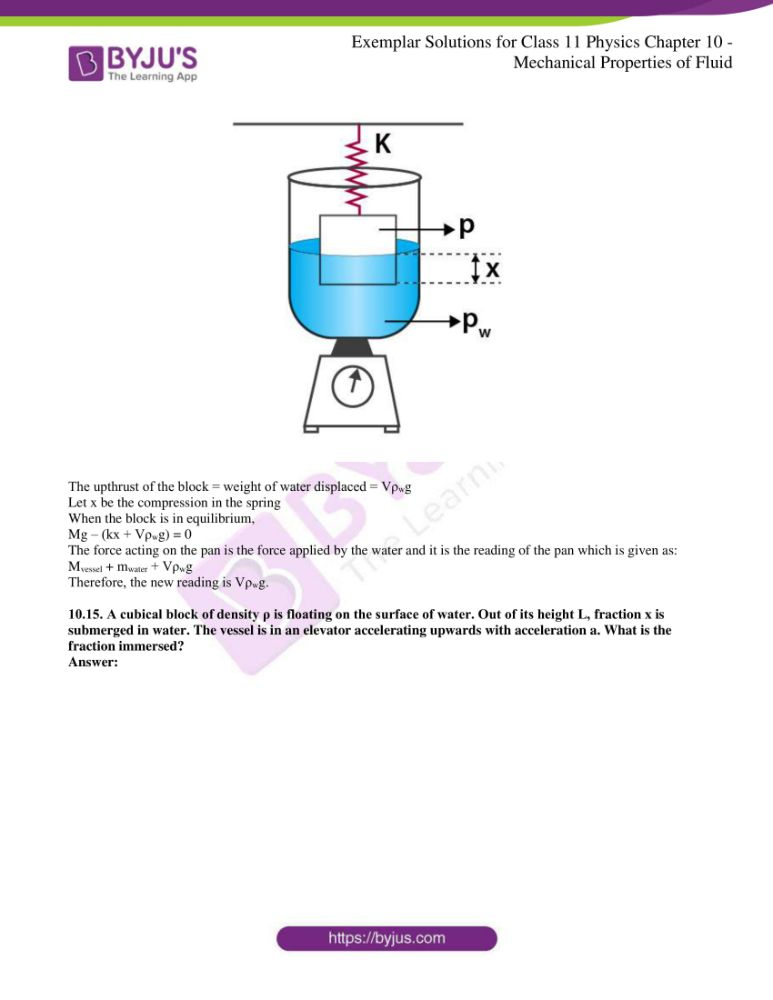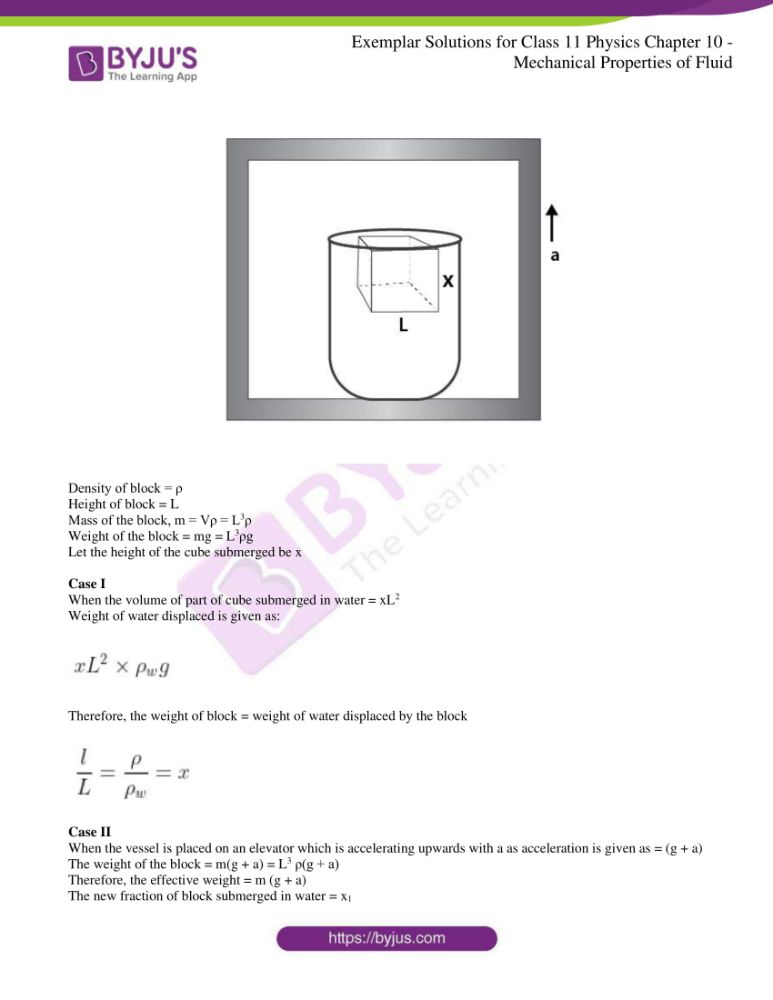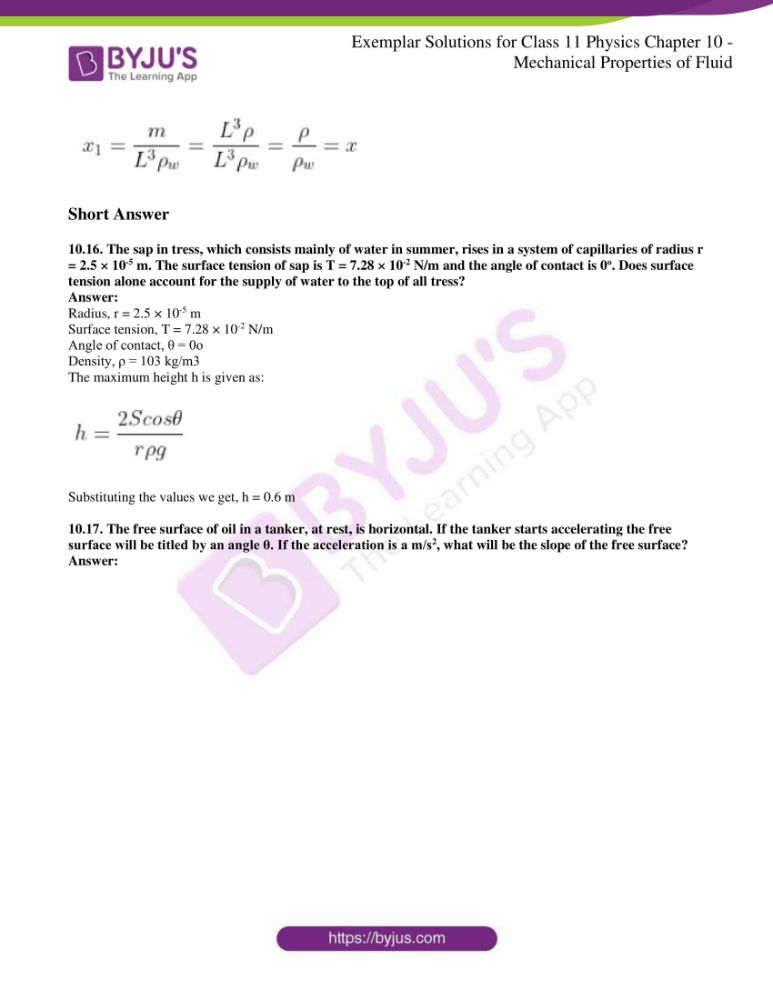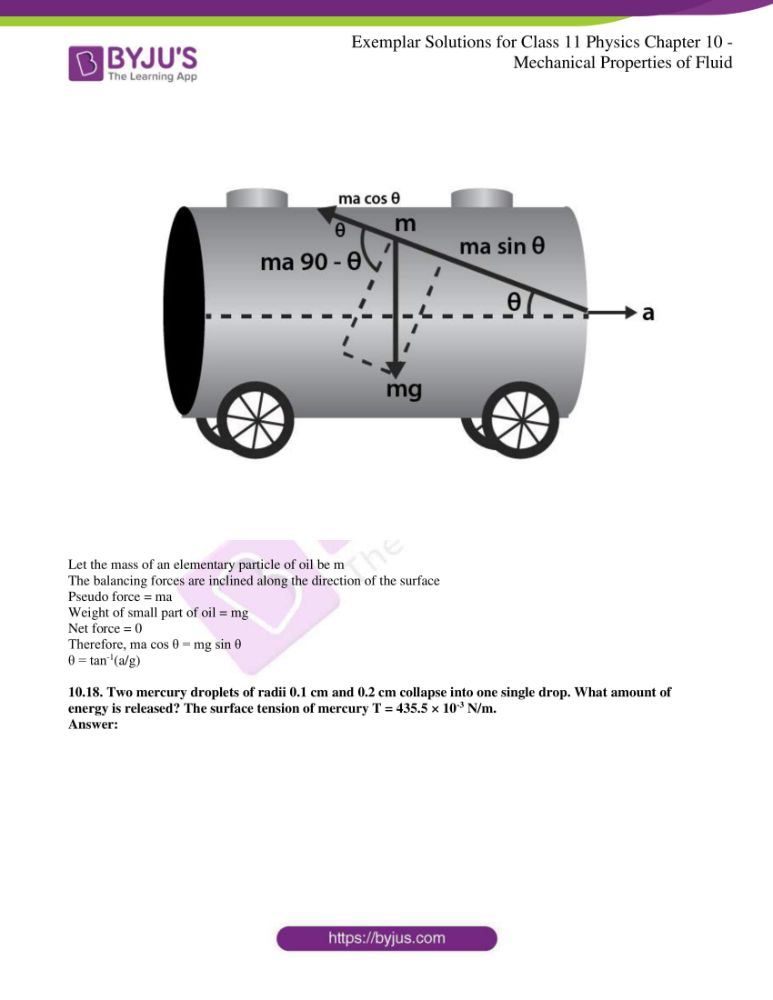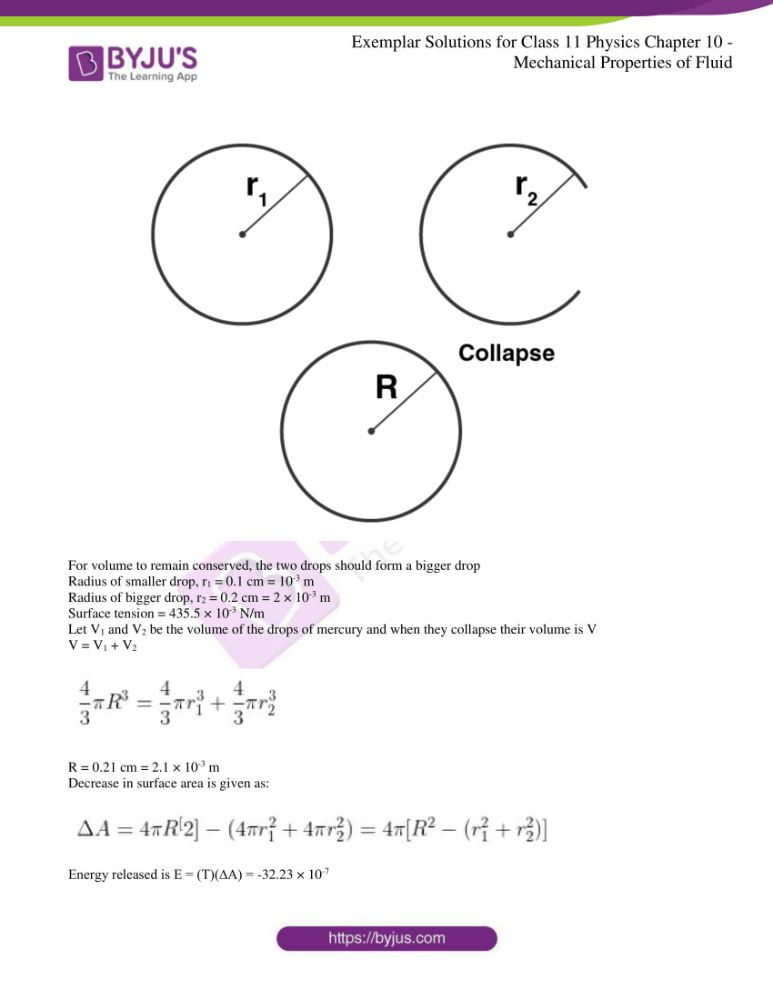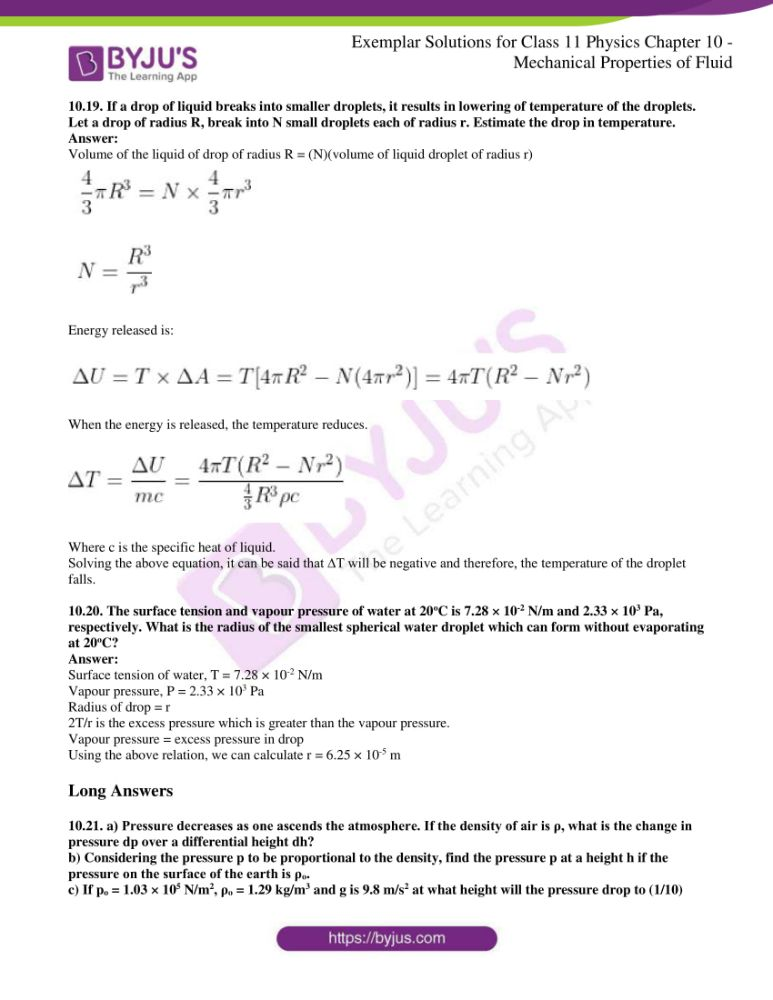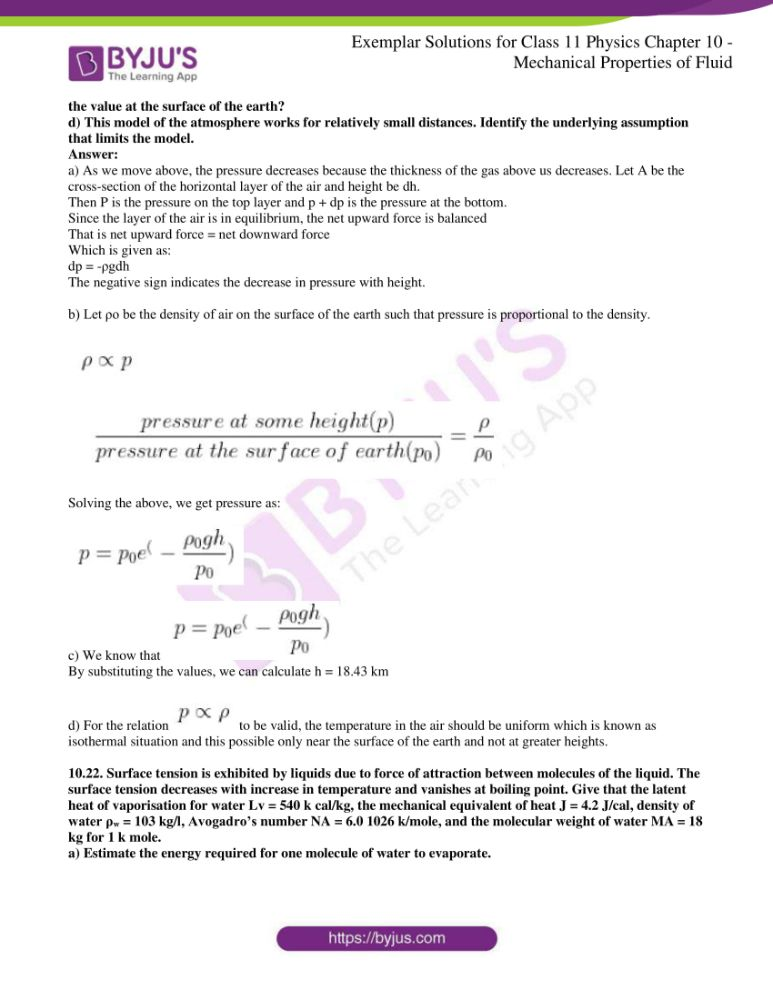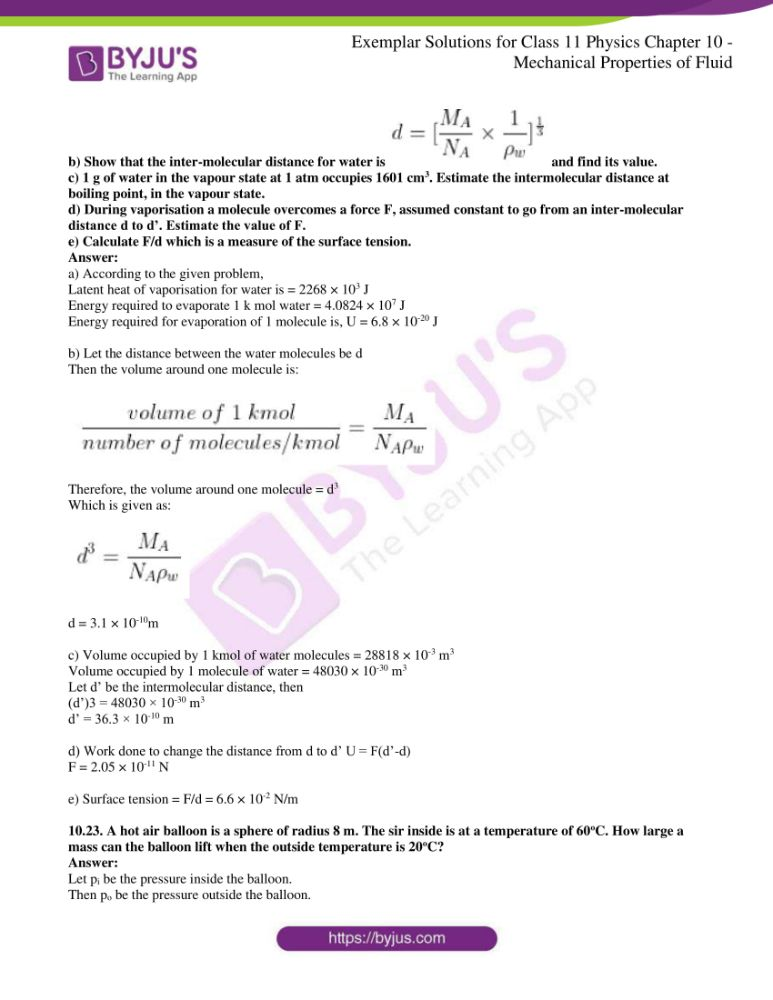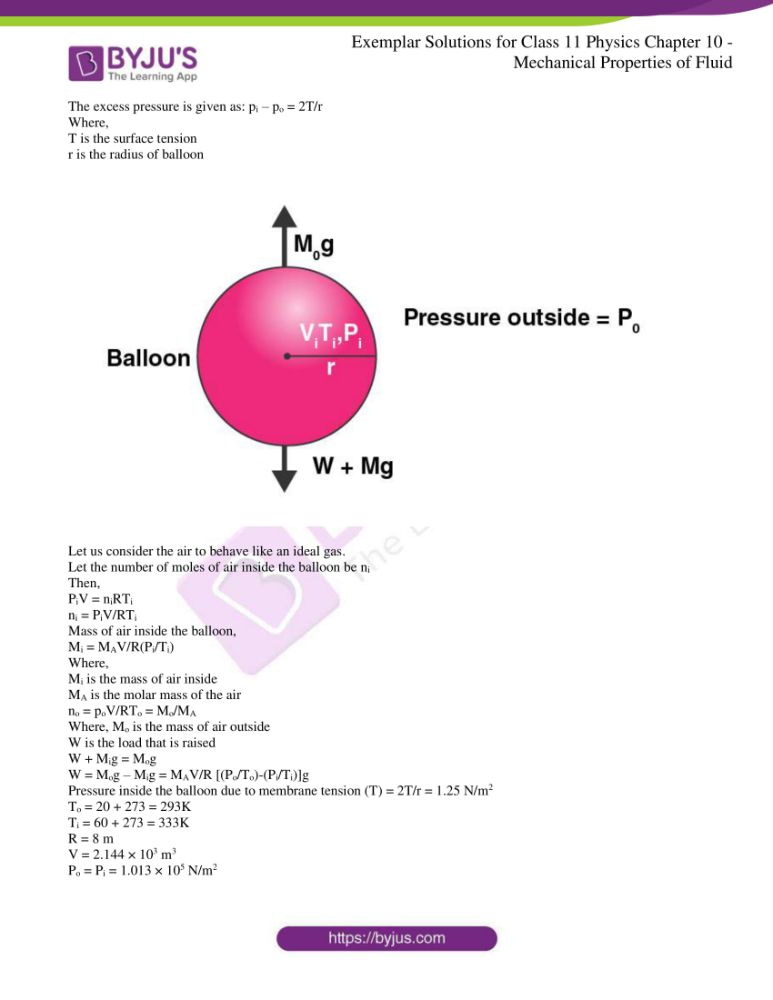### Multiple Choice Questions I

10.1. A tall cylinder is filled with viscous oil. A round pebble is dropped from the top with zero initial velocity. From the plot shown in the figure, indicate the one that represents the velocity (v) of the pebble as a function of time (t).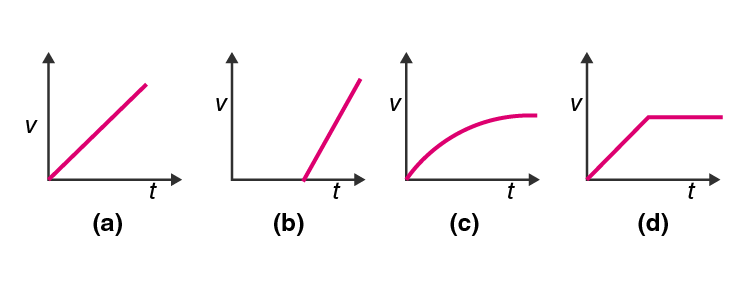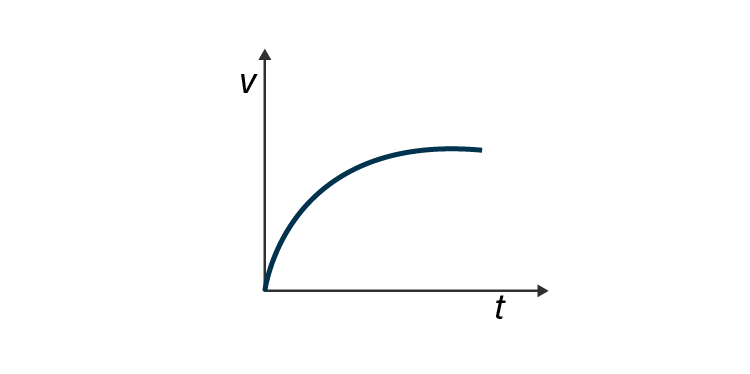10.2. Which of the following diagrams does not represent a streamline flow?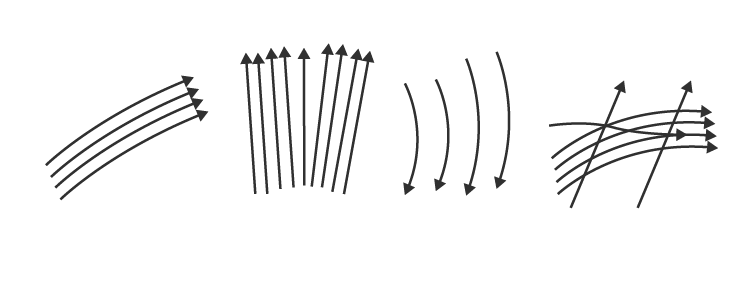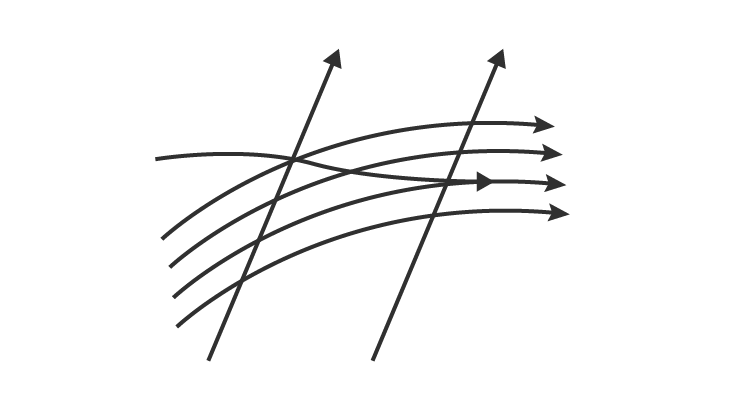10.3. Along a streamline

a) the velocity of a fluid particle remains constant

b) the velocity of all fluid particles crossing a given position is constant

c) the velocity of all fluid particles at a given instant is constant

d) the speed of a fluid particle remains constant

The correct answer is b) the velocity of all particles crossing a given position is constant

10.4. An ideal fluid flows through a pipe of circular cross-section made of two sections with diameters 2.5 cm and 3.75 cm. The ratio of the velocities in the two pipes is

a) 9:4

b) 3:2

c) √3: √2

d) √2: √3

10.5. The angle of contact at the interface of water-glass is 0o, ethyl alcohol-glass is 0o, mercury-glass is 140o, and methyl iodide-glass is 30o. A glass capillary is put in a trough containing one of these four liquids. It is observed that the meniscus is convex. The liquid in the trough is

a) water

b) ethyl alcohol

c) mercury

d) methyl iodide

### Multiple Choice Questions II

10.6. For a surface molecule

a) the net force on it is zero

b) there is a net downward force

c) the potential energy is less than that of a molecule inside

d) the potential energy is more than that of a molecule inside

b) there is a net downward force

d) the potential energy is more than that of a molecule inside

10.7. Pressure is a scalar quantity because

a) it is the ratio of force to the area and both force and area are vectors

b) it is the ratio of the magnitude of the force to area

c) it is the ratio of a component of the force normal to the area

d) it does not depend on the size of the area chosen

b) it is the ratio of the magnitude of the force to area

c) it is the ratio of a component of the force normal to the area

10.8. A wooden block with a coin placed on its top, floats in water as shown in the figure: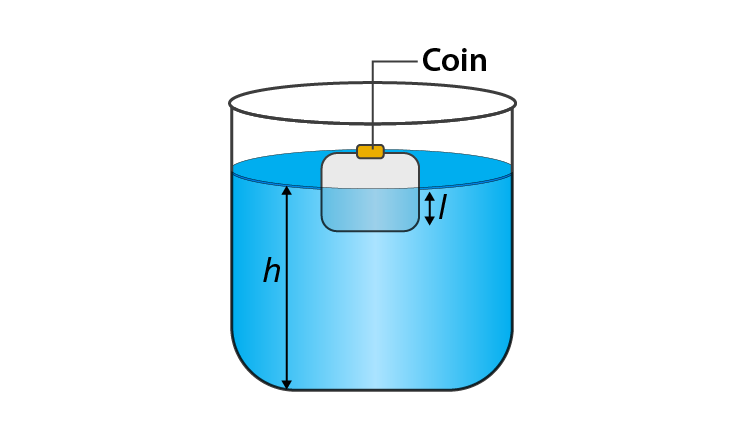The distance l and h are shown in the figure. After some time the coin falls into the water. Then

a) l decreases

b) h decreases

c) l increases

d) h increases

a) l decreases

b) h decreases

10.9. With increase in temperature, the viscosity of

a) gases decreases

b) liquids increases

c) gases increases

d) liquids decreases

c) gases increases

d) liquids decreases

10.10. Streamline flow is more likely for liquids with

a) high density

b) high viscosity

c) low density

d) low viscosity

b) high viscosity

c) low density

10.11. Is viscosity a vector?

Viscosity is not a vector quantity. It is a scalar quantity and it is a property of liquid with no direction.

10.12. Is surface tension a vector?

Surface tension is a scalar quantity as it has a specific direction.

10.13. An iceberg floats in water with part of it submerged. What is the fraction of the volume of an iceberg submerged if the density of ice is ρi = 0.917 g/cm3?

Density of ice, ρice = 0.917 g/cm3

Density of water, ρwater = 1 g/cm3

Vi is the volume of the iceberg

Vw is the volume of the water displaced by the iceberg

Weight of iceberg, W = ρiVig

Upthrust, FB = ρwVwg

At equilibrium, weight of iceberg = Vw/Vi = 0.917

10.14. A vessel filled with water is kept on a weighing pan and the scale adjusted to zero. A block of mass M and density ρ is suspended by a massless spring of spring constant k. This block is submerged inside into the water in the vessel. What is the reading of the scale?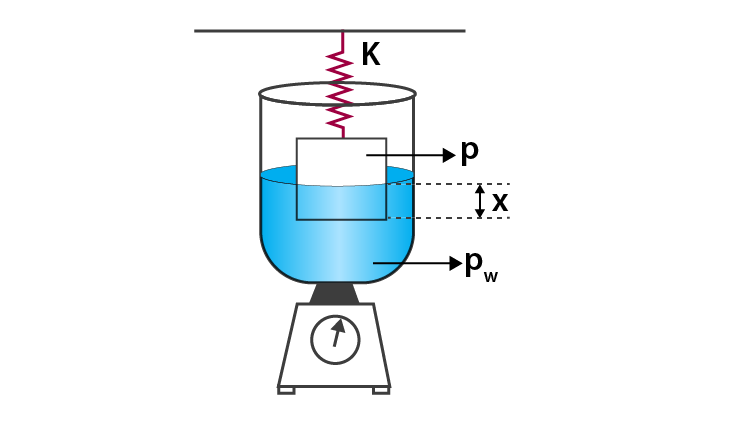The upthrust of the block = weight of water displaced = Vρwg

Let x be the compression in the spring

When the block is in equilibrium,

Mg – (kx + Vρwg) = 0

The force acting on the pan is the force applied by the water and it is the reading of the pan which is given as:

Mvessel + mwater + Vρwg

Therefore, the new reading is Vρwg.

10.15. A cubical block of density ρ is floating on the surface of the water. Out of its height L, fraction x is submerged in water. The vessel is in an elevator accelerating upwards with acceleration a. What is the fraction immersed?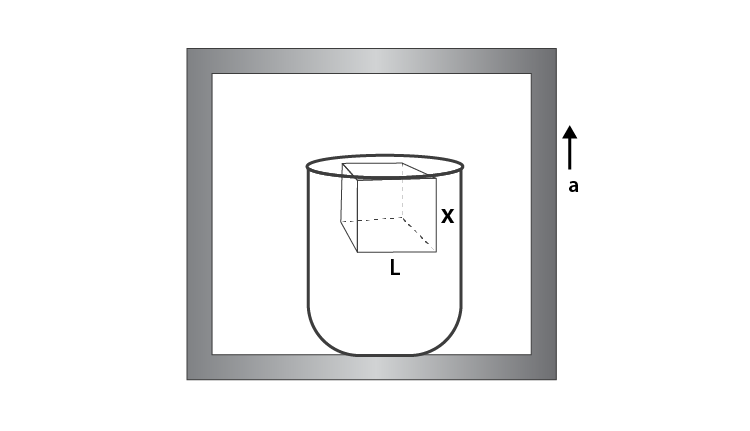Density of block = ρ

Height of block = L

Mass of the block, m = Vρ = L3ρ

Weight of the block = mg = L3ρg

Let the height of the cube submerged be x

Case I

When the volume of part of cube submerged in water = xL2

Weight of water displaced is given as: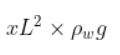Therefore, the weight of block = weight of water displaced by the block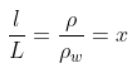Case II

When the vessel is placed on an elevator which is accelerating upwards with a as acceleration is given as = (g + a)

The weight of the block = m(g + a) = L3 ρ(g + a)

Therefore, the effective weight = m (g + a)

The new fraction of block submerged in water = x1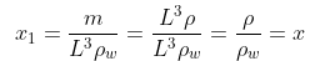10.16. The sap in trees, which consists mainly of water in summer, rises in a system of capillaries of radius r = 2.5 × 10-5 m. The surface tension of sap is T = 7.28 × 10-2 N/m and the angle of contact is 0o. Does surface tension alone account for the supply of water to the top of all tress?

Radius, r = 2.5 × 10-5 m

Surface tension, T = 7.28 × 10-2 N/m

Angle of contact, θ = 0o

Density, ρ = 103 kg/m3

The maximum height h is given as: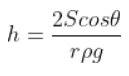Substituting the values we get, h = 0.6 m

10.17. The free surface of the oil in a tanker, at rest, is horizontal. If the tanker starts accelerating the free surface will be titled by an angle θ. If the acceleration is a m/s2, what will be the slope of the free surface?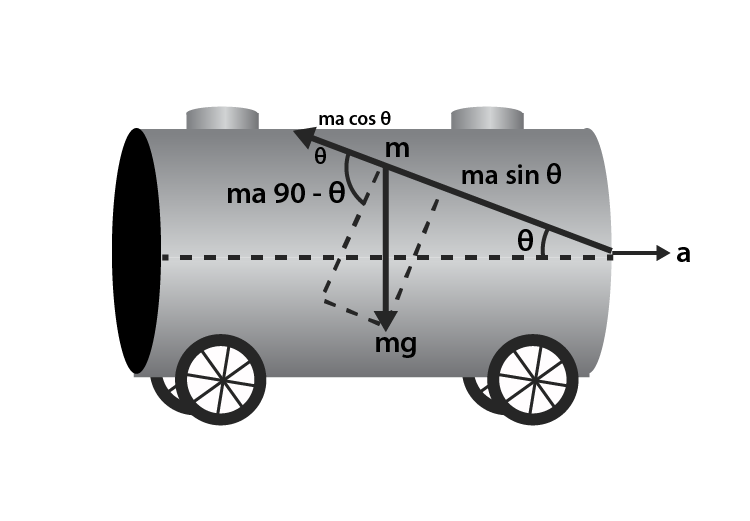Let the mass of an elementary particle of oil be m

The balancing forces are inclined along the direction of the surface

Pseudo force = ma

Weight of small part of oil = mg

Net force = 0

Therefore, ma cos θ = mg sin θ

θ = tan-1(a/g)

10.18. Two mercury droplets of radii 0.1 cm and 0.2 cm collapse into one single drop. What amount of energy is released? The surface tension of mercury T = 435.5 × 10-3 N/m.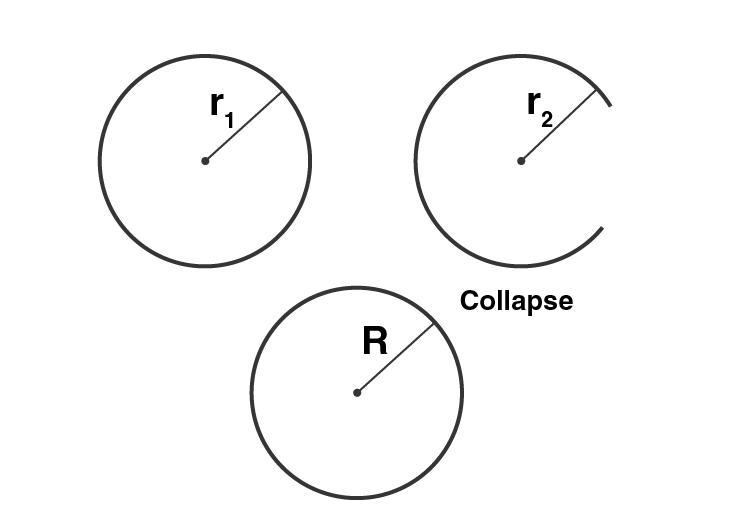For volume to remain conserved, the two drops should form a bigger drop

Radius of smaller drop, r1 = 0.1 cm = 10-3 m

Radius of bigger drop, r2 = 0.2 cm = 2 × 10-3 m

Surface tension = 435.5 × 10-3 N/m

Let V1 and V2 be the volume of the drops of mercury and when they collapse their volume is V

V = V1 + V2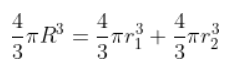R = 0.21 cm = 2.1 × 10-3 m

Decrease in surface area is given as: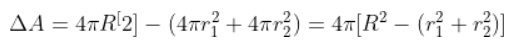Energy released is E = (T)(∆A) = -32.23 × 10-7

10.19. If a drop of liquid breaks into smaller droplets, it results in lowering of the temperature of the droplets. Let a drop of radius R, break into N small droplets each of radius r. Estimate the drop in temperature.

Volume of the liquid of drop of radius R = (N)(volume of liquid droplet of radius r)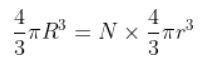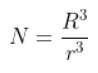Energy released is: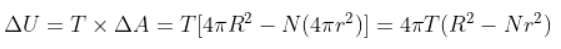When the energy is released, the temperature reduces.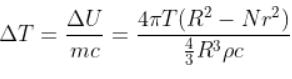Where c is the specific heat of the liquid.

Solving the above equation, it can be said that ∆T will be negative and therefore, the temperature of the droplet falls.

10.20. The surface tension and vapour pressure of water at 20oC is 7.28 × 10-2 N/m and 2.33 × 103 Pa, respectively. What is the radius of the smallest spherical water droplet which can form without evaporating at 20oC?

Surface tension of water, T = 7.28 × 10-2 N/m

Vapour pressure, P = 2.33 × 103 Pa

2T/r is the excess pressure which is greater than the vapour pressure.

Vapour pressure = excess pressure in drop

Using the above relation, we can calculate r = 6.25 × 10-5 m

10.21. a) Pressure decreases as one ascends the atmosphere. If the density of air is ρ, what is the change in pressure dp over a differential height dh?

b) Considering the pressure p to be proportional to the density, find the pressure p at a height h if the pressure on the surface of the earth is ρo.

c) If po = 1.03 × 105 N/m2, ρo = 1.29 kg/m3 and g is 9.8 m/s2 at what height will the pressure drop to (1/10) the value at the surface of the earth?

d) This model of the atmosphere works for relatively small distances. Identify the underlying assumption that limits the model.

a) As we move above, the pressure decreases because the thickness of the gas above us decreases. Let A be the cross-section of the horizontal layer of the air and height be dh.

Then P is the pressure on the top layer and p + dp is the pressure at the bottom.

Since the layer of the air is in equilibrium, the net upward force is balanced

That is net upward force = net downward force

Which is given as:

dp = -ρgdh

b) Let ρo be the density of air on the surface of the earth such that pressure is proportional to the density.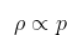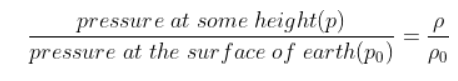Solving the above, we get pressure as: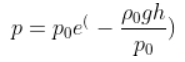c) We know thatBy substituting the values, we can calculate h = 18.43 km

d) For the relation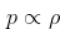to be valid, the temperature in the air should be uniform which is known as isothermal situation and this possible only near the surface of the earth and not at greater heights.

10.22. Surface tension is exhibited by liquids due to the force of attraction between molecules of the liquid. The surface tension decreases with increase in temperature and vanishes at boiling point. Give that the latent heat of vaporisation for water Lv = 540 k cal/kg, the mechanical equivalent of heat J = 4.2 J/cal, density of water ρw = 103 kg/l, Avogadro’s number NA = 6.0 1026 k/mole, and the molecular weight of water MA = 18 kg for 1 k mole.

a) Estimate the energy required for one molecule of water to evaporate.

b) Show that the inter-molecular distance for water is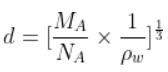and find its value.

c) 1 g of water in the vapour state at 1 atm occupies 1601 cm3. Estimate the intermolecular distance at boiling point, in the vapour state.

d) During vaporisation a molecule overcomes a force F, assumed constant to go from an inter-molecular distance d to d’. Estimate the value of F.

e) Calculate F/d which is a measure of the surface tension.

a) According to the given problem,

Latent heat of vaporisation for water is = 2268 × 103 J

The energy required to evaporate 1 k mol water = 4.0824 × 107 J

The energy required for evaporation of 1 molecule is, U = 6.8 × 10-20 J

b) Let the distance between the water molecules be d

Then the volume around one molecule is: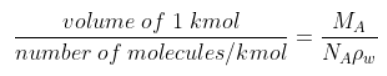Therefore, the volume around one molecule = d3

Which is given as: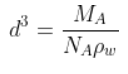d = 3.1 × 10-10m

c) Volume occupied by 1 kmol of water molecules = 28818 × 10-3 m3

Volume occupied by 1 molecule of water = 48030 × 10-30 m3

Let d’ be the intermolecular distance, then

(d’)3 = 48030 × 10-30 m3

d’ = 36.3 × 10-10 m

d) Work done to change the distance from d to d’ U = F(d’-d)

F = 2.05 × 10-11 N

e) Surface tension = F/d = 6.6 × 10-2 N/m

10.23. A hot air balloon is a sphere of radius 8 m. The sir inside is at a temperature of 60oC. How large a mass can the balloon lift when the outside temperature is 20oC?

Let pi be the pressure inside the balloon.

Then po be the pressure outside the balloon.

The excess pressure is given as: pi – po = 2T/r

Where,

T is the surface tension

r is the radius of the balloon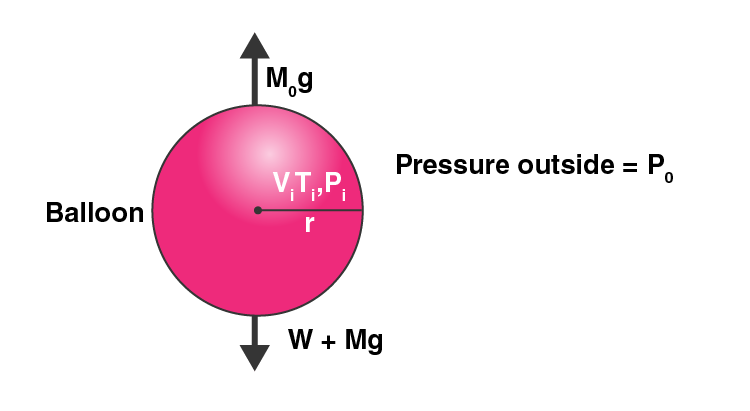Let us consider the air to behave like an ideal gas.

Let the number of moles of air inside the balloon be ni

Then,

PiV = niRTi

ni = PiV/RTi

Mass of air inside the balloon,

Mi = MAV/R(Pi/Ti)

Where,

Mi is the mass of air inside

MA is the molar mass of the air

no = poV/RTo = Mo/MA

Where, Mo is the mass of air outside

W is the load that is raised

W + Mig = Mog

W = Mog – Mig = MAV/R [(Po/To)-(Pi/Ti)]g

Pressure inside the balloon due to membrane tension (T) = 2T/r = 1.25 N/m2

To = 20 + 273 = 293K

Ti = 60 + 273 = 333K

R = 8 m

V = 2.144 × 103 m3

Po = Pi = 1.013 × 105 N/m2

In atmosphere, 21% O2 and 79% N2 is present

Ma = 21% of O2 + 79% of N2 = 28.84 × 10-3 kg/mol

Therefore, the weight of the balloon raised is 301 kg.

### Why to Opt BYJU’s?

Physics experts at BYJU’S provide notes, study materials, worksheets and exercise that help you understand the topic clearly without any difficulty. Understanding the concepts thoroughly will help you in studying the advanced concepts easily. To get access to all the study resources of BYJU’S register with us or Download BYJU’S App for a comprehensive learning experience.